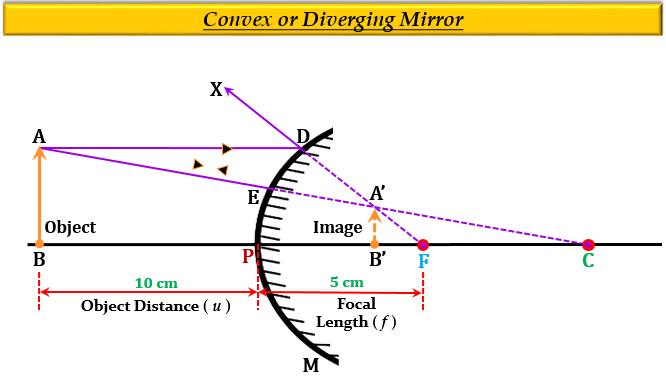# An object is placed at a distance of 10 cm from a convex mirror of focal length 5 cm.(i) Draw a ray-diagram showing the formation image(ii) State two characteristics of the image formed(iii) Calculate the distance of the image from mirror.

(i) Ray Diagram -(ii) The two characteristics of the image formed are:

1. It is virtual and erect. (behind the mirror and upright)

2. It is smaller than the object.

(iii) Given:

Convex Mirror

Distance of the object, $u$ = $-$10 cm

Focal length of the mirror, $f$ = 5 cm

To find: Distance of the image from the mirror, $v$.

Solution:

From the mirror formula, we know that-

$\frac {1}{f}=\frac {1}{v}+\frac {1}{u}$

Substituting the given values we get-

$\frac {1}{5}=\frac {1}{v}+\frac {1}{(-10)}$

$\frac {1}{5}=\frac {1}{v}-\frac {1}{10}$

$\frac {1}{5}+\frac {1}{10}=\frac {1}{v}$

$\frac {1}{v}=\frac {2+1}{10}$

$\frac {1}{v}=\frac {3}{10}$

$v=\frac {10}{3}$

$v=+0.3cm$

Thus, the distance of the image $v$ is 0.3 cm from the mirror, and the positive sign implies that the image forms behind the mirror (on the right).

Updated on: 10-Oct-2022

161 Views